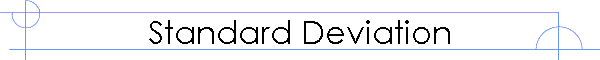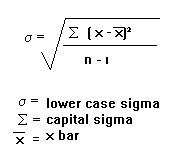Standard DeviationLower case sigma means 'standard deviation'.
Capital sigma means 'the sum of'.
x bar means 'the mean'

The standard deviation measures the spread of the data about the mean value. It is useful in comparing sets of data which may have the same mean but a different range. For example, the mean of the following two is the same: 15, 15, 15, 14, 16 and 2, 7, 14, 22, 30. However, the second is clearly more spread out. If a set has a low standard deviation, the values are not spread out too much.

Example:
Find the standard deviation of 4, 9, 11, 12, 17, 5, 8, 12, 14
First work out the mean: 10.222
Now, subtract the mean individually from each of the numbers in the question and square the result. This is equivalent to the (x - xbar)ý step. x refers to the values in the question.

x               4        9        11       12       17       5       8       12      14
(x - x)ý     38.7   1.49    0.60    3.16    45.9   27.3   4.94    3.16   14.3

Now add up these results (this is the 'sigma' in the formula): 139.55
Divide by n-1. n is the number of values, so in this case is 8: 17.44
And finally, square root this: 4.18

The standard deviation can usually be calculated much more easily with a calculator and this is usually acceptable in exams. With some calculators, you go into the standard deviation mode (often mode '.'). Then type in the first value, press 'data', type in the second value, press 'data'. Do this until you have typed in all the values, then press the standard deviation button (it will probably have a lower case sigma on it). Check your calculator's manual to see how to calculate it on yours.